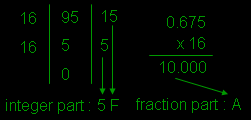Next generation website theme is here (black, neon and white color) - Reduces eye strain and headache problems.

## Decimal Number System

Represents 10 types of digits from 0 to 9, so the base of the number system is 10. This is the most familiar number system with everyone. Similar to other number system, value of digit depends upon the position occupied in the number.

### Decimal to all conversion

Decimal to binary conversion

Steps:

• Divide the number by two and keep track of the remainder.
• Stop when quotient reaches to zero.
• Arrange these remainders horizontally from bottom to top.
• For fraction part it is multiplied by 2 and keeping track of integers generated.
• Arrange these integers as they are generated.

Example:

Convert (38.625)10 into binary(38.625)10 = (100110.101)2

Decimal to octal conversion

Steps:

• Same as decimal to binary conversion but it is divided by 8 to integer part and multiplied by 8 to fractional part.

Example:

Convert (180.6875)10 to octal(180.6875)10 = (264.54)8

Decimal to hexadecimal conversion

Steps:

• Same as above but divide by 16 for integer part and multiply by 16 to fractional part.

Example:

Convert (90.675)10 to hexadecimal(90.675)10 = (5F.A)16

+

## Tip Box

I don't think you need a tip on decimal number system, as you know it.

## Advertisement

CodesandTutorials © 2014 All Rights Reserved